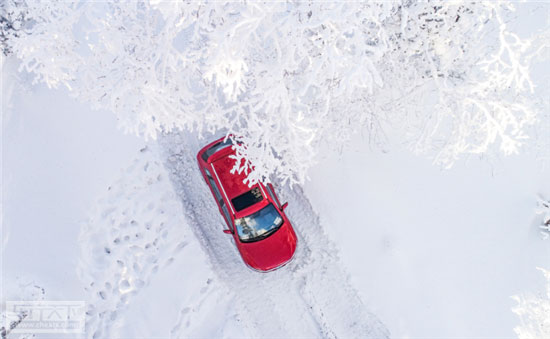# 一汽吉林 森雅R7

## 一汽森雅R7挑战严寒 驭战中国雪乡

2016年12月20日 09:38 来源：www.chextx.com 超过：13186次关注

雪乡，素有“天无三日晴之说”，冬季气温一般都在-20℃以下，年平均积雪厚度达2米，雪量堪称中国之最。12月17日，森雅R7“柒待·玩美之行”冰雪试驾活动就在这里以凌寒斗雪的豪情蓬勃展开。试驾活动中，森雅R7自动挡车型穿越苍莽雪原，向全国媒体展示了其在零下20度冰天雪地中的卓越品质，充分彰显出其在专业化、高性能高安全以及人性化三个层面上的品质新跨越。在-20℃严寒中，钢铁、橡胶、塑料都会变脆，一些平时看上去不起眼的颠簸、磕碰，可能会造成断开、破裂；手机、相机等电子产品也会暂时“罢工”。可以说，严寒用车环境是对整车品质的全面考验，直接体现厂家的专业化造车水准。本次雪乡冰雪试驾，便见证了森雅R7在行业内领先的专业化实力。众所周知，我国地域辽阔，气候、路况等用车环境复杂多样，对此森雅R7以比肩主流合资车企的严苛管理标准确保其产品的全面高品质。它不仅严格采用中国一汽的自主研发创新体系，充分利用一汽集团的全球研发资源；同时，还充分吸收和借鉴精益化管理方式，提高装配质量，为全面高品质打造奠定了坚实基础。其核心产品组件均由全球精英供应商提供，安全系统源自世界级安全设备供应商“博世”、全车铰链设备源于专业铰链供应商“尼特固”、自动挡变速箱来自“爱信精机”，国际级供应商阵容，共同打造了卓越的品质。

值得一提的是，投产之前，森雅R7还进行了动力、爆晒、涉水等性能试验；高速、山路、强化坏路等场地可靠性试验；以及寒带、热带、高原、风沙、典型地区等区域适应性试验，总里程达500万公里，相当于绕地球赤道125圈。性能与安全是汽车设计与制造的永恒话题，每年举办的“冰雪达喀尔”汽车越野赛，便是众多车企展现其产品高性能与高安全的角逐舞台。而本次森雅R7自动挡车型的雪乡试驾，虽然无法与“冰雪达喀尔”相提并论，但其从亚布力到二浪河44公里的长途奔袭，以及从二浪河到中国雪乡40公里的复杂冰雪穿越，则可堪称模拟版“冰雪达喀尔”，使森雅R7自动挡车型高性能、高安全的产品特点，在驭战冰雪中显露无遗。在冰雪湿滑路面行驶中刹车过猛、加速太急或转向不足、过度，易导致车辆侧滑、甩尾等现象发生。森雅R7搭载了7位一体智能主动安全系统，该系统包含ABS+EBD、ESP、牵引力控制、刹车辅助、上坡辅助等。在车辆转向、过弯道的时候，该系统可及时保持车身的稳定，有效防止打滑、甩尾、跑偏等意外的发生。除此，森雅R7还采用纯正的SUV底盘设计，以及麦弗逊独立前悬架和扭力梁式半独立后悬架，能有效过滤路面的颠簸，实现舒适与运动的平衡，保证车辆的安全性和稳定性。“不管冰天雪地，胜似闲庭信步”，越级的舒适配置让森雅R7在这次雪乡严寒挑战中，平添人性化的温暖，焕发品质魅力。

森雅R7自动挡车型配有6向调节驾驶座椅并搭配腰部支撑及电加热，充分为本次冰雪试驾提供舒适保障。它还配有自动空调，后排足部设计了采暖出风口，在室内温控舒适性上效果突出，充分体现了对后排冬季乘坐的人性化考量。同时，它还配有“外后视镜电加热”功能，为此次试驾带来安全的行车视野。

而大家熟悉的360°全景影像、9寸大屏、车内氛围灯、无钥匙进入+一键启动、天窗、定速巡航、发动机怠速启停等同级领先配置也一应俱全，真正做到了人无我有，人有我优，满足消费者对新潮科技的探索与追求。除此，森雅R7自动挡车型还搭载爱信第三代6AT手自一体变速器，换挡平顺，让我们能够尽情的征战冰雪。在本次试驾活动中，森雅R7自动挡车型很好地经受住了严寒环境的考验。不管是产品的软硬件配置还是带给我们的驾驶感受，它都展现出了过硬的品质，也刷新了我们对于中国自主品牌车型的传统认知。古诗有云：“已是悬崖百丈冰，犹有花枝俏”，相信森雅R7自动挡雪乡试驾的精彩诗篇，将为一汽自主品牌带来盎然春意。

#### 相关文章

0-500 字已有评论 0条 查看评论>>

### 森雅R7### 猜你喜欢

﻿
• 快速找车
• 选择品牌
• 选择品牌
• A  奥迪
• A  阿斯顿·马丁
• A  阿尔法·罗密欧
• B  宝沃
• B  布加迪
• B  巴博斯
• B  保时捷
• B  宾利
• B  奔驰
• B  宝马
• B  本田
• B  别克
• B  标致
• B  比亚迪
• B  宝骏
• B  北汽制造
• B  北汽新能源
• B  北汽幻速
• B  北汽威旺
• B  北京汽车
• B  奔腾
• B  北汽绅宝
• C  长安
• C  长安商用
• C  长城
• C  昌河
• D  大众
• D  道奇
• D  DS
• D  东南
• D  东风风神
• D  东风风行
• D  东风小康
• D  东风风度
• D  东风
• F  福特
• F  丰田
• F  菲亚特
• F  法拉利
• F  福田
• F  福迪
• F  福汽启腾
• G  观致
• G  广汽传祺
• G  广汽吉奥
• G  GMC
• H  红旗
• H  汉腾汽车
• H  哈弗
• H  哈飞
• H  海格
• H  海马
• H  华颂
• H  黄海
• H  华泰
• H  恒天
• J  吉利汽车
• J  捷豹
• J  Jeep
• J  江淮
• J  江铃
• J  金杯
• J  九龙
• J  金旅
• K  凯翼
• K  凯迪拉克
• K  克莱斯勒
• K  科尼塞克
• K  卡威
• K  开瑞
• L  路虎
• L  林肯
• L  劳斯莱斯
• L  兰博基尼
• L  雷克萨斯
• L  铃木
• L  雷诺
• L  理念
• L  力帆
• L  莲花汽车
• L  猎豹
• L  路特斯
• L  陆风
• M  马自达
• M  MG
• M  MINI
• M  玛莎拉蒂
• M  摩根
• M  迈凯轮
• N  纳智捷
• O  欧宝
• O  讴歌
• O  欧朗
• Q  奇瑞
• Q  起亚
• Q  启辰
• R  日产
• R  荣威
• R  瑞麒
• S  三菱
• S  斯威汽车
• S  萨博
• S  smart
• S  斯柯达
• S  斯巴鲁
• S  思铭
• S  双龙
• S  上汽大通
• S  双环
• T  特斯拉
• T  腾势
• W  沃尔沃
• W  五菱汽车
• W  五十铃
• W  威兹曼
• W  威麟
• X  现代
• X  雪佛兰
• X  雪铁龙
• X  西雅特
• Y  一汽
• Y  英菲尼迪
• Y  英致
• Y  依维柯
• Y  野马汽车
• Y  永源
• Z  众泰
• Z  中华
• Z  中兴
• Z  知豆
• 选择车系
• 选择车系
• 车型对比
• 选择品牌
• 选择品牌
• A  奥迪
• A  阿斯顿·马丁
• A  阿尔法·罗密欧
• B  宝沃
• B  布加迪
• B  巴博斯
• B  保时捷
• B  宾利
• B  奔驰
• B  宝马
• B  本田
• B  别克
• B  标致
• B  比亚迪
• B  宝骏
• B  北汽制造
• B  北汽新能源
• B  北汽幻速
• B  北汽威旺
• B  北京汽车
• B  奔腾
• B  北汽绅宝
• C  长安
• C  长安商用
• C  长城
• C  昌河
• D  大众
• D  道奇
• D  DS
• D  东南
• D  东风风神
• D  东风风行
• D  东风小康
• D  东风风度
• D  东风
• F  福特
• F  丰田
• F  菲亚特
• F  法拉利
• F  福田
• F  福迪
• F  福汽启腾
• G  观致
• G  广汽传祺
• G  广汽吉奥
• G  GMC
• H  红旗
• H  汉腾汽车
• H  哈弗
• H  哈飞
• H  海格
• H  海马
• H  华颂
• H  黄海
• H  华泰
• H  恒天
• J  吉利汽车
• J  捷豹
• J  Jeep
• J  江淮
• J  江铃
• J  金杯
• J  九龙
• J  金旅
• K  凯翼
• K  凯迪拉克
• K  克莱斯勒
• K  科尼塞克
• K  卡威
• K  开瑞
• L  路虎
• L  林肯
• L  劳斯莱斯
• L  兰博基尼
• L  雷克萨斯
• L  铃木
• L  雷诺
• L  理念
• L  力帆
• L  莲花汽车
• L  猎豹
• L  路特斯
• L  陆风
• M  马自达
• M  MG
• M  MINI
• M  玛莎拉蒂
• M  摩根
• M  迈凯轮
• N  纳智捷
• O  欧宝
• O  讴歌
• O  欧朗
• Q  奇瑞
• Q  起亚
• Q  启辰
• R  日产
• R  荣威
• R  瑞麒
• S  三菱
• S  斯威汽车
• S  萨博
• S  smart
• S  斯柯达
• S  斯巴鲁
• S  思铭
• S  双龙
• S  上汽大通
• S  双环
• T  特斯拉
• T  腾势
• W  沃尔沃
• W  五菱汽车
• W  五十铃
• W  威兹曼
• W  威麟
• X  现代
• X  雪佛兰
• X  雪铁龙
• X  西雅特
• Y  一汽
• Y  英菲尼迪
• Y  英致
• Y  依维柯
• Y  野马汽车
• Y  永源
• Z  众泰
• Z  中华
• Z  中兴
• Z  知豆
• 选择车系
• 选择车系
• 选择车型
• 选择车型
• 意见反馈